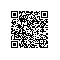# JavaScript 函数

## JavaScript 函数定义

JavaScript 使用关键字 function 定义函数

### 函数声明

function sum1(n1,n2){
return n1+n2;
};

### 函数表达式，又叫函数字面量

JavaScript 函数可以通过一个表达式定义

var sum2=function(n1,n2){
return n1+n2;
};

var x = function (a, b) {return a * b};
var z = x(4, 3);

### 函数的几种写法

1 常见写法:

function Test(){
}
Test()

2 匿名函数：

var test=function(){
}
test();

3 自调用函数：

(function () {
})();

4 函数嵌套:

var Test = {
test1:function(){
},
test2:function(){
}
}
var t = Test.test1();

5 函数继承和构造函数

var Test = function(){};
Test.prototype={
test1:function(){
},test2:function(){
}
}
var t = new Test();
t.test2();

### Function() 构造函数

var myFunction = new Function("a", "b", "return a * b");
var x = myFunction(4, 3);

var myFunction = function (a, b) {return a * b}
var x = myFunction(4, 3);

### 函数提升（Hoisting）

 myFunction(5);
function myFunction(y) {
return y * y;
} 

 myFunction(5);
var myFunction = function(y) {
return y * y;
} 

### 自调用函数

(function () {
var x = "Hello!!";      // 我将调用自己
})();

var sum=(function(x,y){
return x+y;
})(2,3);
console.log(sum);

#### JavaScript函数可作为一个值使用

function myFunction(a, b) {
return a * b;
}
var x = myFunction(4, 3);

#### JavaScript函数可作为表达式使用：

function myFunction(a, b) {
return a * b;
}
var x = myFunction(4, 3) * 2;

### 函数是对象

JavaScript 函数有属性和方法。

arguments.length 属性返回函数调用过程接收到的参数个数：

function myFunction(a, b) {
return arguments.length;
}
document.getElementById("demo").innerHTML = myFunction(4,3,6,5);

toString() 方法将函数作为一个字符串返回:

## JavaScript 函数参数

JavaScript 函数对参数的值(arguments)没有进行任何的检查

### 函数显式参数与隐藏参数(arguments)

functionName(parameter1, parameter2, parameter3) {
code to be executed
}

### 参数规则

JavaScript 函数定义时参数没有指定数据类型,对隐藏参数(arguments)没有进行检测,对隐藏参数(arguments)的个数没有进行检测

### 默认参数

function myFunction(x, y) {
if (y === undefined) {
y = 0;
}
}

function myFunction(x, y) {
y = y || 0;
}

### Arguments 对象

JavaScript 函数有个内置的对象 arguments 对象。arguments 对象包含了函数调用的参数数组。通过这种方式你可以很方便的找到每个参数的值：

x = findMax(1, 123, 500, 115, 44, 88);
function findMax() {
var i, max = 0;
for (i = 0; i < arguments.length; i++) {
if (arguments[i] > max) {
max = arguments[i];
}
}
return max;
}

x = sumAll(1, 123, 500, 115, 44, 88);
function sumAll() {
var i, sum = 0;
for (i = 0; i < arguments.length; i++) {
sum += arguments[i];
}
return sum;
}

### 通过值传递参数

var x = 1;
function myFunction(x) {// 通过值传递参数
x++; //修改参数x的值，将不会修改在函数外定义的变量 x
console.log(x);
}
myFunction(x); // 2
console.log(x); // 1

### 通过对象传递参数

var obj = {x:1};
function myFunction(obj) {// 通过对象传递参数
obj.x++; //修改参数对象obj.x的值，函数外定义的obj也将会被修改
console.log(obj.x);
}
myFunction(obj); // 2
console.log(obj.x); // 2

## JavaScript 函数调用

JavaScript 函数有 4 种调用方式。每种方式的不同方式在于 this 的初始化

### 调用 JavaScript 函数

#### 作为一个函数调用

function myFunction(a, b) {
return a * b;
}
myFunction(10, 2);           // myFunction(10, 2) 返回 20

myFunction() 和 window.myFunction() 是一样的：

function myFunction(a, b) {
return a * b;
}
window.myFunction(10, 2);    // window.myFunction(10, 2) 返回 20

##### 全局对象

function myFunction() {
return this;
}
myFunction();                // 返回 window 对象

#### 函数作为方法调用

var myObject = {
firstName:"John",
lastName: "Doe",
fullName: function () {
return this.firstName + " " + this.lastName;
}
}
myObject.fullName();         // 返回 "John Doe"

fullName 方法是一个函数。函数属于对象。 myObject 是函数的所有者。

this对象，拥有 JavaScript 代码。实例中 this 的值为 myObject 对象。

var myObject = {
firstName:"John",
lastName: "Doe",
fullName: function () {
return this;
}
}
myObject.fullName();          // 返回 [object Object](所有者对象)

#### 使用构造函数调用函数，参数必须加引号

// 构造函数:
function myFunction(arg1, arg2) {
this.firstName = arg1;
this.lastName  = arg2;
}
// This creates a new object
var x = new myFunction("John","Doe");
x.firstName;                             // 返回 "John"

#### 作为函数方法调用函数

//call()
function myFunction(a, b) {
return a * b;
}
myFunction.call(myObject, 10, 2);      // 返回 20

//apply()
function myFunction(a, b) {
return a * b;
}
myArray = [10,2];
myFunction.apply(myObject, myArray);   // 返回 20

## JavaScript 闭包

JavaScript 变量可以是局部变量或全局变量

### 局部变量和全局变量

function myFunction() {
var a = 4;
return a * a;
}

var a = 4;
function myFunction() {
return a * a;
}

### 计数器困境

var counter = 0;
counter += 1;
}
// 计数器现在为 3

function add() {
var counter = 0;
counter += 1;
}
// 本意是想输出 3, 但事与愿违，输出的都是 1 !

### JavaScript 内嵌函数

function add() {
var counter = 0;
function plus() {counter += 1;}
plus();
return counter;
}

### JavaScript 闭包

var add = (function () {
var counter = 0;
return function () {return counter += 1;}
})();
// 计数器为 3使用钉钉扫一扫加入圈子
+ 订阅